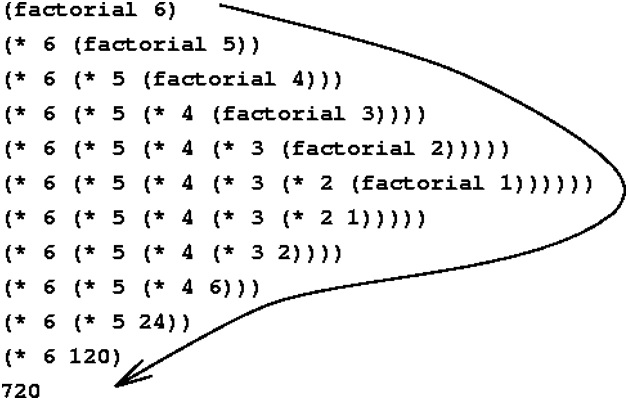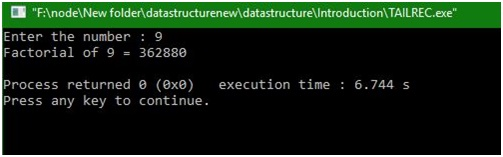# Find the factorial of any number with the use of tail recursion

In this article we are going to learn how to use tail recursion and also implement it to find the factorial of the number?
Submitted by Manu Jemini, on January 13, 2018

## What is factorial?

Factorial can be understood as the product of all the integers from 1 to n, where n is the number of which we have to find the factorial of.

Example:

```    Input number: 5
Output:
Factorial is: 120
Explanation: 1 * 2 * 3 *4 * 5 = 120
```

You may have noticed that the operation always remain same or we can say that its business logic never changes. Taking this into the account we can think about which approach to choose. You can do this with looping but we have chosen Recursion. Now what recursion refers to is like an approach to the flow of data and control?

When a function calls itself, again and again, it said to be a recursion.## C program for factorial using recursion

```#include<stdio.h>

//function that will return factorial
//this function will be executed recursively
int factorial( int n, int fact )
{
if ( n==1 )
return fact;
else
factorial( n-1, n*fact );
}

//main function to test above function
int main( ){
int n,value;

//input an integer number
printf( "Enter the number : " );
scanf( "%d", &n );

if ( n < 0 )
printf( "No factorial of negative number\n" );
else if ( n==0 )
printf( "Factorial of  zero is 1\n" );
else
{
value = factorial( n,1 ); /* Function for factorial of number */
printf( "Factorial of %d = %d\n",n,value );
}

return 0;
}
```

Output